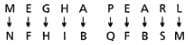# Coding And Decoding

## 10 Questions MCQ Test Science Olympiad Class 4 | Coding And Decoding

Description
Attempt Coding And Decoding | 10 questions in 20 minutes | Mock test for Class 4 preparation | Free important questions MCQ to study Science Olympiad Class 4 for Class 4 Exam | Download free PDF with solutions
QUESTION: 1

Solution:
QUESTION: 2

Solution:
QUESTION: 3

### If MEGHA is coded as NFHIB and PEARL is coded as QFBSM, then ​Identify the code for PRABHA.

Solution:
QUESTION: 4

If MEGHA is coded as NFHIB and PEARL is coded as QFBSM, then
​Identify the code for AKHILESH.

Solution:
QUESTION: 5

If MEGHA is coded as NFHIB and PEARL is coded as QFBSM, then
​Identify the code for FAMILY.

Solution:
QUESTION: 6

If VRUNDA is coded as XTWPFC and SWEETU is coded as UYGGVW, then
Identify the code for RAKESH.

Solution:
QUESTION: 7

If VRUNDA is coded as XTWPFC and SWEETU is coded as UYGGVW, then
​Identify the code for VARSHA.

Solution:
QUESTION: 8

If VRUNDA is coded as XTWPFC and SWEETU is coded as UYGGVW, then
Identify the code for THERMAL.

Solution:
QUESTION: 9

If VRUNDA is coded as XTWPFC and SWEETU is coded as UYGGVW, then
Identify the code for CHINTU.

Solution:
QUESTION: 10

If VRUNDA is coded as XTWPFC and SWEETU is coded as UYGGVW, then
Identify the code PINTU.

Solution:

The code for PINTU is RKPVW. Each letter in the word is moved one step forward to obtain the corresponding letter of the code.Use Code STAYHOME200 and get INR 200 additional OFF Use Coupon Code